## 5.1.4 Using the Correct Measure

Since many metrics and measures are possible, it may sometimes seem that there is no correct'' choice. This can be frustrating because the performance of sampling-based planning algorithms can depend strongly on these. Conveniently, there is a natural measure, called the Haar measure, for some transformation groups, including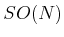. Good metrics also follow from the Haar measure, but unfortunately, there are still arbitrary alternatives.

The basic requirement is that the measure does not vary when the sets are transformed using the group elements. More formally, let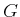represent a matrix group with real-valued entries, and let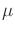denote a measure on. If for any measurable subset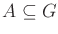, and any element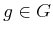,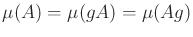, thenis called the Haar measure5.2 for. The notation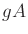represents the set of all matrices obtained by the product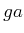, for any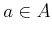. Similarly,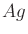represents all products of the form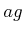.

Example 5..13 (Haar Measure for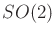)   The Haar measure forcan be obtained by parameterizing the rotations as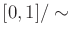with 0 andidentified, and lettingbe the Lebesgue measure on the unit interval. To see the invariance property, consider the interval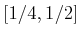, which produces a set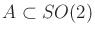of rotation matrices. This corresponds to the set of all rotations from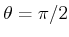to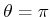. The measure yields. Now consider multiplying every matrixby a rotation matrix,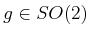, to yield. Suppose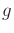is the rotation matrix for. The setis the set of all rotation matrices from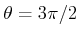up to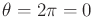. The measure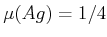remains unchanged. Invariance formay be checked similarly. The transformationtranslates the intervals in. Since the measure is based on interval lengths, it is invariant with respect to translation. Note thatcan be multiplied by a fixed constant (such as) without affecting the invariance property.

An invariant metric can be defined from the Haar measure on. For any points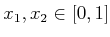, let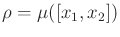, in which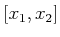is the shortest length (smallest measure) interval that contains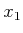and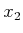as endpoints. This metric was already given in Example 5.2.

To obtain examples that are not the Haar measure, letrepresent probability mass over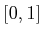and define any nonuniform probability density function (the uniform density yields the Haar measure). Any shifting of intervals will change the probability mass, resulting in a different measure.

Failing to use the Haar measure weights some parts ofmore heavily than others. Sometimes imposing a bias may be desirable, but it is at least as important to know how to eliminate bias. These ideas may appear obvious, but in the case of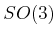and many other groups it is more challenging to eliminate this bias and obtain the Haar measure.Example 5..14 (Haar Measure for)   Forit turns out once again that quaternions come to the rescue. If unit quaternions are used, recall thatbecomes parameterized in terms of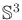, but opposite points are identified. It can be shown that the surface area onis the Haar measure. (Sinceis a 3D manifold, it may more appropriately be considered as a surface volume.'') It will be seen in Section 5.2.2 that uniform random sampling overmust be done with a uniform probability density over. This corresponds exactly to the Haar measure. If insteadis parameterized with Euler angles, the Haar measure will not be obtained. An unintentional bias will be introduced; some rotations inwill have more weight than others for no particularly good reason.Steven M LaValle 2020-08-14